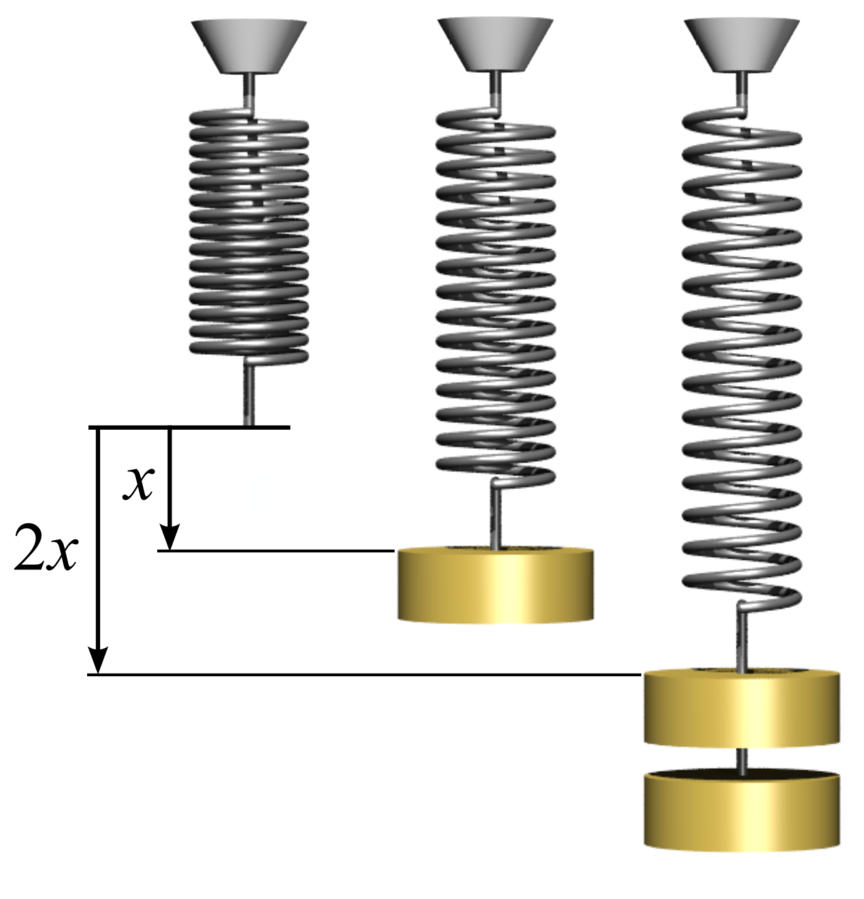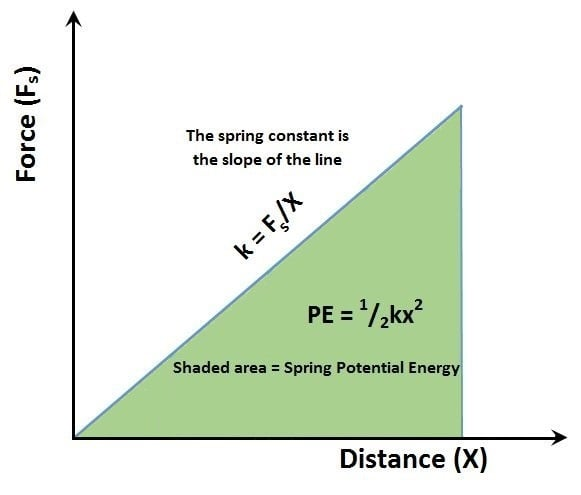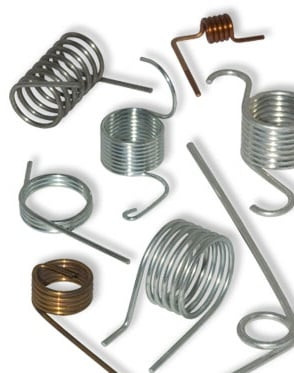A spring is one of the simplest devices for supplying mechanical energy. Springs are made of a material such as spring steel that returns to its original shape after being compressed, stretched or twisted.

When deformed from its free state, the spring stores energy in the form of elastic potential energy, which releases when the spring is freed. How much energy is stored in the spring and how much force does it take to deform it?

## Brief History of Springs

The earliest spring usage was limited to non-coiled springs. The bow (from a bow and arrow) is a simple spring that discharges potential energy when released. Several examples of metal springs first appeared in the Bronze Age. Roman tweezers made of bronze are an example of an early metal spring application. Chinese inventors became the first to develop spring locks containing a flat spring. The Egyptians used flat springs to create a basic chariot suspension system.

The next evolution in spring design was spiral springs, whose invention dates to the early 15th century. Initial applications included door locks and clocks. By the 16th century, spiral spring use in larger watches started to appear.Robert Hooke. Source: Biography.comIn 1676, British scientist Robert Hooke discovered the law of elasticity, which states that a spring exerts a force proportional to the distance it is extended or compressed. This is now known as Hooke’s Law. Hooke described his discovery as “ut tension, sic vis,” which translates to “as the extension, so the force.” Hooke also developed the balance spring, which allowed watches to keep accurate time.

It wasn’t until 1763 that British citizen Richard Tradwell invented and obtained Great Britain Patent 792, titled “Springs for Carriages,” for the first coil spring. Although intended for the suspension of a carriage, the springs in his patent never appeared in that application. Other spring inventions started to appear around the same time.

Spring technology continued to develop through the Industrial Revolution with material breakthroughs as well as innovations in spring manufacturing equipment. Spring winding machines brought mass production capabilities, which made springs an affordable commodity for a variety of applications. Today, springs can be found in watches, printers, machine tools, computers, automobiles, bicycles, toys, pens, mechanical pencils, flashlights and more. Springs are virtually everywhere.

When selecting a coil spring for an application, one specification an engineer has to determine is the amount of potential energy the spring must supply. Using data regarding the spring material, size and deformation distance, the energy can easily be calculated.

According to Hooke’s Law, the force to compress or extend a spring a specified distance is proportional to that distance.

## Extension and Compression SpringsHooke's Law: the force is proportional to the distance. Source: Svjo / CC BY-SA 3.0 The spring constant (k) is typically supplied by the spring manufacturer, but it can be calculated if unknown. There are two formulas for determining the spring constant of compression and extension springs.

Method 1:

Use this method if the load and the distance traveled are known.

Fs = kx

where F = force (N or lbf), x = distance (mm or in), k = spring constant (N/mm or lbf/in)

The spring constant (k) is a measure of the spring's stiffness. Since the force is directly proportional to the distance the spring is deformed, it follows that it takes two times as much force to compress a spring twice the distance.

The spring constant is usually supplied by the spring manufacturer, but can easily be calculated using the formula above by moving the spring with a known force and measuring the deformation. Several different factors affect the stiffness of a spring, including the spring material, wire thickness, wire diameter and spring length.

k = Fs/x

Method 2:

Use this method if you know the spring dimensions, but do not know the load or distance traveled.

k = Gd4/8D3N

where G = shear modulus of material in torsion, d = wire size (mm or in), D = mean diameter (mm or in), N = number of active coils

The shear modulus in torsion (also referred to as the modulus of rigidity) is the ratio of the shear stress to the shear strain and is one of the measures used to determine the material stiffness. It is a property of the material and cannot be changed. The shear modulus for common spring wires is shown below.

• Music Wire – 11.5 psi x 10^6 (79.3 MPa x 10^3)
• Stainless Steel – 11.2 psi x 10^6 (77.2 MPa x 10^3)
• Phosphor Bronze – 5.9 psi x 10^6 (41 MPa x 10^3)
• Monel – 9.6 psi x 10^6 (66 MPa x 10^3)
• Inconel – 11.5 x 10^6 (79 MPa x 10^3)
• Copper – 6.5 x 10^6 (45 MPa x 10^3)
• Beryllium Copper – 6.9 x 10^6 (48 MPa x 10^3)Source: IEEE GlobalSpecOnce the spring constant is determined, calculating the potential energy is easy. Calculate the elastic potential energy of a spring with the following formula:

PE = 1/2kx2

Example 1:

Using method 1 from above to calculate the spring constant.

If you hang a 0.5 kg mass on the end of the extension spring and it moves 10 mm from its free state with no weight, what is the spring constant?

Since we know the mass and the distance moved, we can use method #1 above for calculating the spring constant. The first thing that needs to be done is to convert the mass to a force, and then plug this into the spring constant formula.

F = ma, where m = mass and a = acceleration due to gravity (32.2 ft/s2 or 9.81 m/s2)

F = (0.5 kg)(9.81 m/s2)
F = 4.90 N

Now the force and distance can be entered into the spring constant formula.

k = Fs/x

k = (4.90 N)/(10 mm)
k = (4.90 N)/(0.01 m)
k = 490.5 N/m

Now that the spring constant is known, the potential energy can be calculated using the formula above.

If the spring in the example above is extended 20 mm from the equilibrium position, the potential energy can be calculated as follows:

PE = 1/2kx2

PE = 1/2(490.5 N/m)(20 mm)2
PE = 1/2(490.5 N/m)(0.02 m)2
PE = 0.098 N-m

PE = 0.098 joule

Example 2:

Using method #2 from above to calculate the spring constant.

Note: Examples 1 and 2 use different specifications and answers are not intended to match.

Material = music wire, wire diameter (d) = 0.062, D = 0.375, outside diameter (OD) = 0.500, number of active coils (N) = 7

k = Gd4/8D3N

k = (11.5 x 106 psi)(0.062 in)4/[(8)(0.375 in)3(7)]
k = 169.93 lb-in2/2.95 in3
k = 57.54 lb/in

If the spring in the example above is extended 0.75 in from the equilibrium position, the potential energy can be calculated as follows:

PE = 1/2kx2

PE = 1/2(57.54 ib/in)(0.75 in)2
PE = 16.18 in-lb
PE = 1.35 ft-lb

## Torsion SpringsSource: Century SpringCalculating the potential energy of a torsion spring is very similar to that of compression and extension springs.

The spring constant for a torsion spring is calculated differently and it is critical that this is done correctly. As with compression and extension springs, most manufacturers will supply the spring constant, avoiding this calculation. The spring rate for a torsion spring is defined as the amount of force per 360 degrees of travel. To perform the calculation, you need four pieces of information.

• E = Young’s modulus of elasticity (psi or MPa)
• d = wire diameter (in or mm)
• D = mean diameter (in or mm)
• Na = number of active coils

Young’s modulus of elasticity is a property measuring a material’s stiffness and is defined as the stress divided by the strain. It was named after Thomas Young, a 19th-century British scientist. Every material has a Young’s Modulus that cannot be changed.

Young’s Modulus = Stress/Strain where Stress = Force/(Cross-sectional area)

Strain = (Change in length)/Original length)

The modulus of elasticity for common spring wires is shown below.

• Music Wire – 30 psi x 10^6 (207 MPa x 10^3)
• Stainless Steel – 28 psi x 10^6 (193 MPa x 10^3)
• Chrome Vanadium – 30 psi x 10^6 (207 MPa x 10^3)
• Chrome Silicon – 30 psi x 10^6 (207 MPa x 10^3)
• Phosphor Bronze – 15 psi x 10^6 (103 MPa x 10^3)

The formula for calculating the spring constant per 360 degrees (R) is:

(R) = Ed4/10.8 DNa with the units of lbf-in/degree or N-m/degree

where E = Modulus of Elasticity, d = wire diameter (in or mm), D = mean diameter (in or mm), Na = number of active coils

Once the spring rate is known, the potential energy can be calculated in a manner similar to compression and expression springs.

PE = 1/2kq 2 where q is the angle of twist in radians

The torsion force, or torque, of the spring, can be determined from the spring constant the distance traveled.

Load = (Rate per degree)(Distance traveled)

Example:

Spring specifications:

Material = music wire (E = 30 psi), wire diameter (d) = .038 in, mean diameter (D) = 0.547 in, number of active coils (Na) = 3.5, q = twist of 90 degrees (1.57 radians)

(R) = Ed4/10.8 DNa

(R) = ((30 psi x 106)(.038 in)4)/[(10.8)(0.547 in)(3.5)]
(R) = 62.554 lb-in2 / 20.677 in
(R) = 3.025 in-lb /(360 degrees) or 0.0084 in-lb/degree or 0.482 in-lb/radian

Plug this into the potential energy calculation above to get:

PE = 1/2kq 2